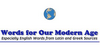### multiplyThe act of multiplication, represented by a x in a sum.
Example: 5 x 2 = 10
Found on http://www.bbc.co.uk/skillswise/glossary/

### Multiply• (v. i.) To increase amount of gold or silver by the arts of alchemy. • (v. t.) To add (any given number or quantity) to itself a certain number of times; to find the product of by multiplication; thus 7 multiplied by 8 produces the number 56; to multiply two numbers. See the Note under Multiplication. • (v. i.) To increase in exten...
Found on http://thinkexist.com/dictionary/meaning/multiply/

### multiply1. To become greater in number; to become numerous. 'When men began to multiply on the face of the earth, and daughters were born unto them.' (Gen. Vi. 1) ... 2. To increase in extent and influence; to spread. 'The word of God grew and multiplied.' (Acts xii. 24) ... 3. To increase amount of gold or silver by the arts of alchemy. ... 1. To increase...
Found on http://www.encyclo.co.uk/local/20973

### multiplyadverb in several ways; in a multiple manner; `they were multiply checked for errors`
Found on https://www.encyclo.co.uk/local/20974

### Multiply[website] Multiply was a social networking service with an emphasis on allowing users to share media – such as photos, videos and blog entries – with their `real-world` network. The website was launched in March 2004 and is privately held with backing by VantagePoint Venture Partners, Point Judith Capital, Transcosmos, and private inves...
Found on http://en.wikipedia.org/wiki/Multiply_(website)

### MultiplyMul'ti·ply intransitive verb 1. To become greater in number; to become numerous. « When men began to multiply on the face of the earth, and daughters were born unto them.» Gen. vi. 1. 2. To increase in extent and influence; to spread. « The word of G...
Found on http://www.encyclo.co.uk/webster/M/114

### MultiplyMul'ti·ply transitive verb [ imperfect & past participle Multiplied ; present participle & verbal noun Multiplying .] [ French multiplier , Latin multiplicare , from multiplex manifold. See Multitude , C...
Found on http://www.encyclo.co.uk/webster/M/114

### multiplymultiply 1. To perform the mathematical operation of multiplication. 2. To increase or make something increase by a considerable number, amount, or degree. 3. To increase in number by breeding.
Found on http://www.wordinfo.info/words/index/info/view_unit/1701/5

### multiply[adv] - in several ways 2. [v] - combine by multiplication 3. [v] - combine or increase by multiplication
Found on http://www.webdictionary.co.uk/definition.php?query=multiply
No exact match found